CUET  >  Waves MCQ Level - 1

# Waves MCQ Level - 1 - CUET

Test Description

## 10 Questions MCQ Test General Test Preparation for CUET - Waves MCQ Level - 1

Waves MCQ Level - 1 for CUET 2023 is part of General Test Preparation for CUET preparation. The Waves MCQ Level - 1 questions and answers have been prepared according to the CUET exam syllabus.The Waves MCQ Level - 1 MCQs are made for CUET 2023 Exam. Find important definitions, questions, notes, meanings, examples, exercises, MCQs and online tests for Waves MCQ Level - 1 below.
Solutions of Waves MCQ Level - 1 questions in English are available as part of our General Test Preparation for CUET for CUET & Waves MCQ Level - 1 solutions in Hindi for General Test Preparation for CUET course. Download more important topics, notes, lectures and mock test series for CUET Exam by signing up for free. Attempt Waves MCQ Level - 1 | 10 questions in 30 minutes | Mock test for CUET preparation | Free important questions MCQ to study General Test Preparation for CUET for CUET Exam | Download free PDF with solutions
 1 Crore+ students have signed up on EduRev. Have you?
Waves MCQ Level - 1 - Question 1

### The equation of a wave disturbance is given as :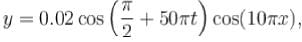where X and y are in metres and t in seconds. Choose the wrong statement:

Detailed Solution for Waves MCQ Level - 1 - Question 1

y=0.02cos(π/2+50π∗t)cos(10π∗x)

At x=0.3m
y=0.02cos(π/2+50π∗t)cos(3π) = −0.02cos(π/2+50π∗t) (It is right)

Now compare the given equation by the standard stationary wave equation,

y=Acos(ϕ+ω∗t)cos(2π/λ∗x)

2π/λ=10π, λ=0.2m (it is also right)

At x=0.15m,

y=0.02cos(π/2+50π∗t)cos(π/2),

and

v=Aω=0.02∗50π=πms−1

so option d is incorrect.

Waves MCQ Level - 1 - Question 2

### In a plane progressive harmonic wave particle speed is always less than the wave speed if: (where A is wavelength of wave)

Detailed Solution for Waves MCQ Level - 1 - Question 2

Let equation of a plane progressive harmonic wave be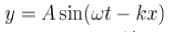wave speed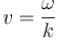and maximum particle speed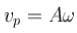Given that vp < v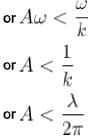The Correct answer is : amplitude of wave is less than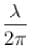Waves MCQ Level - 1 - Question 3

### Which of the following is not the standard form of a sine wave?

Detailed Solution for Waves MCQ Level - 1 - Question 3

Standard form of a sine wave is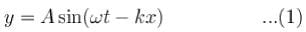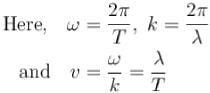With these substitutions we can see that Equation (1) can be written as options (b).
The correct answer is: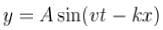Waves MCQ Level - 1 - Question 4

Two sound source are moving in opposite directions with velocities v1 and v2(v1 > v2). Both are moving away from a stationary observer. The frequency of both the sources is 900 Hz. What is the value of v1 - v2 so that the beat frequency observed by the observer is 6 Hz. Speed of sound v = 300 mis. Given, that v1 and v2 « v.

Detailed Solution for Waves MCQ Level - 1 - Question 4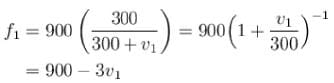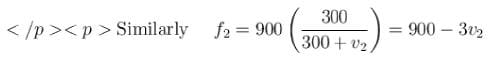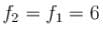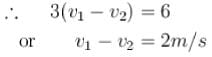The correct answer is: 2 m/s

Waves MCQ Level - 1 - Question 5

String 1 has twice the length, twice the radius, twice the tension and twice the density of another string 2. The relation between the fundamental frequencies of 1 and 2 is :

Detailed Solution for Waves MCQ Level - 1 - Question 5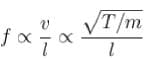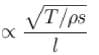(m = mass per unit length =pS)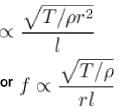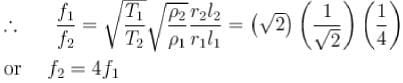The correct answer is: f2 = 4f1

Waves MCQ Level - 1 - Question 6

A string of length L is stretched by L/20 and speed of transverse wave along it is v. The speed of wave when it is stretched by L/10 will be : (assume the Hooke’s law is applicable)

Detailed Solution for Waves MCQ Level - 1 - Question 6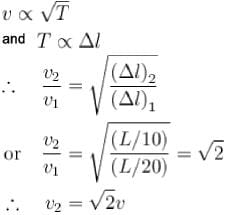Waves MCQ Level - 1 - Question 7

Speed of transverse wave in a string of density 100 kglm3 and area of cross-section 10 mm2 under the tension of 103 N is :

Detailed Solution for Waves MCQ Level - 1 - Question 7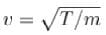Here, m = mass per unit length = pS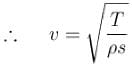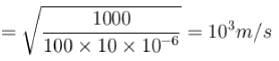The correct answer is: 1000 m/s

Waves MCQ Level - 1 - Question 8

A wave represented by the equation y1 = Acos(kx- ωt), is superimposed with another wave to form a stationary wave such that the point x = 0 is a node. The equation of the other wave is :

Detailed Solution for Waves MCQ Level - 1 - Question 8

y=y1 + y2
At x = 0, y = 0 for all values of t only when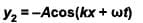The correct answer is: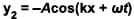Waves MCQ Level - 1 - Question 9

The frequency changes by 10% as the source approaches a stationary observer with constant speed vs. What would be the percentage change in frequency as the source recedes the observer with the same speed ? Given, that vs « v ( v = speed of sound in air)

Detailed Solution for Waves MCQ Level - 1 - Question 9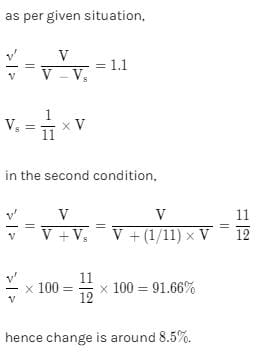Waves MCQ Level - 1 - Question 10

A heavy rope is suspended from a rigid support. A wave pulse is set up at the lower end, then :

Detailed Solution for Waves MCQ Level - 1 - Question 10

Due to weight of the rope, the tension will increase along the rope from the lower end to the upper end. Hence, the pulse will travel with increasing speed as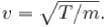The correct answer is: the pulse will travel with increasing speed

## General Test Preparation for CUET

176 videos|328 docs|399 tests
Information about Waves MCQ Level - 1 Page
In this test you can find the Exam questions for Waves MCQ Level - 1 solved & explained in the simplest way possible. Besides giving Questions and answers for Waves MCQ Level - 1, EduRev gives you an ample number of Online tests for practice

## General Test Preparation for CUET

176 videos|328 docs|399 tests